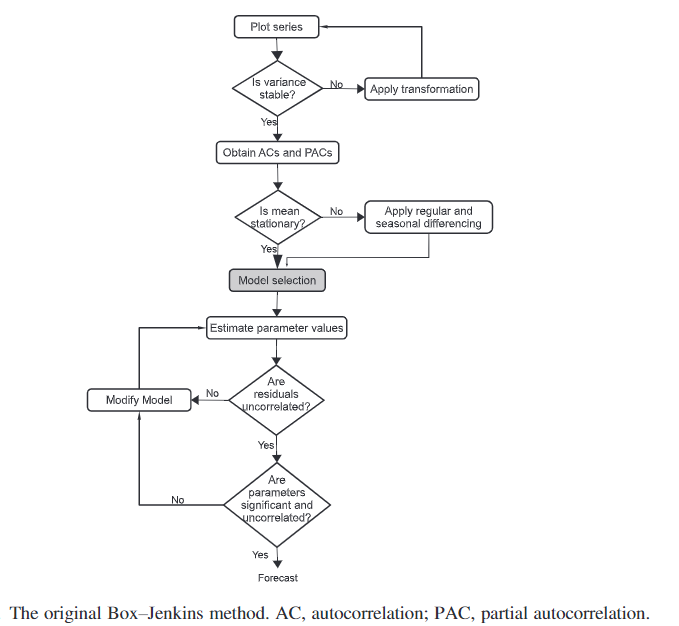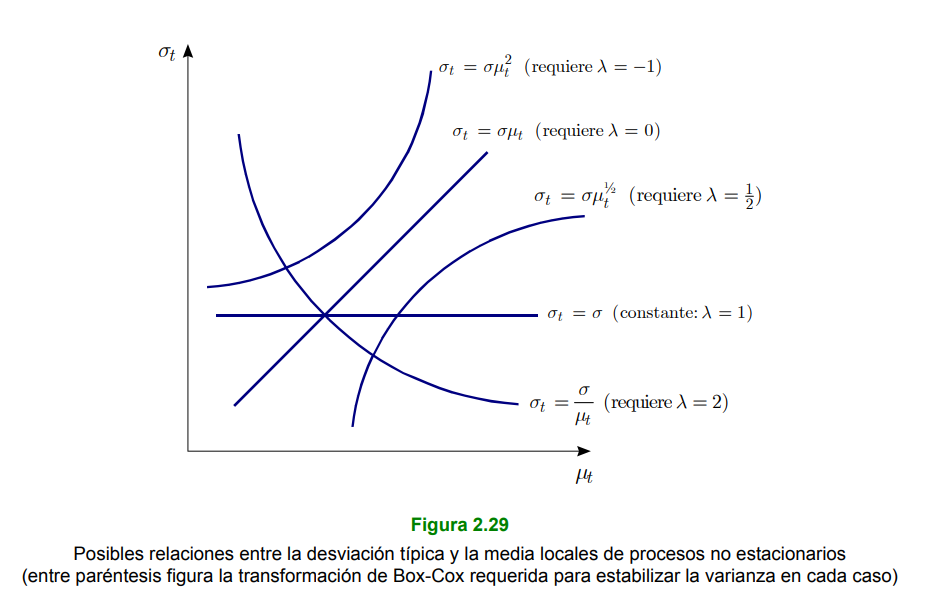# 21 Non-Stationary Stochastic Processes

A stochastic process $(Y_{t}right)$ is non-stationary when the statistical properties of at least one finite sequence $Y_{t_{1}}}, Y_{t_{2}}, , Y_{t_{n}}(n )$ of components of $(Y_{t}right)$, are different from those of the sequence $$Y_{t_{1}+h}, Y_{t_{2}+h}, \ldots, Y_{t_{n}+h}$$ for at least one integer $$h&gt;0$$.Many time series cannot be considered to be generated by stationary stochastic processes (i.e., they are non-stationary series), because:

• they show some clear trend in their time evolution (so that they do not show affinity towards some constant value over time),
• because their dispersion is not constant, because they are seasonal, or for various combinations of these reasons

However, many non-stationary time series can be transformed in a suitable way to obtain stationary-looking series, which can be used as a starting point to build $$operatorname{ARMA}(p, q)$$ models in practice.

To convert a non-stationary series into a stationary one, two types of transformations are usually employed in practice: a first type to stabilize its dispersion (i.e. to induce variance stationarity) and a second type to stabilize its level (i.e. to eliminate its trend or to induce mean stationarity).

### 21.0.1 Non-stationarity in Variance

Let $$\left(Y_{t}\right)$$ a non-stationary stochastic process such that

$\mu_{t} \equiv \mathrm{E}\left[Y_{t}\right] \, \text { and } \, \sigma_{t}^{2} \equiv \operatorname{Var}\left[Y_{t}\right]$ existen y dependen de $$t$$ (por lo que no son constantes). Si

$\sigma_{t}^{2}=\sigma^{2} \times h\left(\mu_{t}\right)^{2}$

where $^{2}>0$ is a constant and $h()$ is a real function such that $h()$ for any value of ${t}$, then a variance-stabilizing transformation of $(Y{t}right)$ is any real function $$g(\cdot)$$ such that $g^{prime}()=$ for any value of $_{t} .$

#### 21.0.1.1 Dispersion Stabilization - Logarithmic Transformation

When the dispersion of a non-stationary time series $Y_{t}(t=1, , N)$ is not constant because its local standard deviation is approximately proportional to its local level, the dispersion of the log-transformed series ${t}=Y{t}(t=1, , N)$ is usually reasonably stable.### 21.0.2 Non-stationarity in Mean

A stochastic process $(Y_{t}right)$ is integrated of order $d(d$ integer), or $()$, if and only if the process $(^{d} Y_{t}right)$ follows a $(p, q)$ model that is stationary and invertible. In such a case, it is usually written

$\left(Y_{t}\right) \sim \mathrm{I}(d)$.

#### 21.0.2.1 Mean Level Stabilization (average) General - Regular Differentiation

The regular difference operator of order $d(d .$ integer) is represented by the symbol $$\nabla^{d}$$ $$\left(\right.$$ sometimes $$\left.\Delta^{d}\right)$$ and is defined as $$\nabla^{d} \equiv(1-B)^{d}$$ (where $$B$$ is the lad operator), so such that

$\nabla X_{t} \equiv X_{t}-X_{t-1}, \nabla^{2} X_{t} \equiv X_{t}-2 X_{t-1}+X_{t-2}, \ldots$

where $$X_{t}$$ is a variable (real or random) relative to a given time $$t$$.

#### 21.0.2.2 Deseasonalization - Seasonal Differentiation

The seasonal difference operator of period $$S$$ and order $$D(D \geq 1$$ integer) is represented by the symbol ${S}^{D}$ (sometimes $. {S}^{D} )$ and is defined as $_{S}^{D} (1-B^{S} )^{D}$ (where $$B$$ is the delay operator), so that, in particular (when $$D=1$$ ),

$\nabla_{S} X_{t} \equiv X_{t}-X_{t-S}$ where $$X_{t}$$ is a variable (real or random) relative to a given time $$t$$.